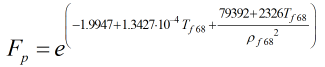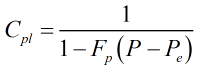# C250 – API MPMS 2004: Calculation of Cpl

## Description

This calculates the correction factor due to pressure.

API MPMS Chapter 11.1 – Temperature and Pressure Volume Correction Factors for Generalized Crude Oils, Refined Products, and Lubricating Oils (ASTM D 1250-04; IP 200/04) (First edition 2004, Addendum 1 2007)

Kelton calculation reference C250

This calculation is used by:

F060/K214 – API Density Referral 2004

## Options

### Thermal Expansion Coefficient

• User Entered

Select this option if you know the thermal expansion factor at 60°F of the hydrocarbon you are performing calculations on. You will not need to select a commodity group if you select this option.

• Calculated

Select this option if you do not know the thermal expansion factor at 60°F of the hydrocarbon you are performing calculations on.

### Commodity Group

• Crude Oil
• Fuel Oils
• Jet Fuels
• Transition Zone
• Gasoline
• Lubricating Oil

Select appropriate commodity so that the correct K values are used in the calculation. They are needed to calculate the thermal expansion factor of the liquid.

## Constants

Constants as defined by API

 Commodity group K0 K1 K2 Crude oil 341.0957 0 0 Fuel oils 103.8720 0.2701 0 Jet fuels 330.301 0 0 Transition Zone 1489.067 0 -0.0018684 Gasolines 192.4571 0.2438 0 Lubricating oil 0 0.34878 0

## Calculation

### Scaled compressibility factor

The scaled compressibility factor, Fp (in psi-1), is calculated by:Where Tf68 = Temperature shifted to ITPS-68 basis ρf68 = Base density shifted to ITPS-68 basis

### Pressure correction factor

The pressure correction factor, Cpl, is calculated by:Where Fp = Scaled compressibility Factor P = Pressure Pe = Vapour pressure

Back to FLOCALC Calculations